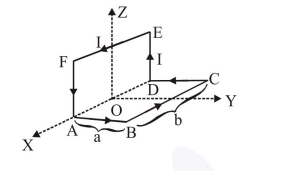# A wire carrying current I is bent in the shape ABCDEFA

Question:

A wire carrying current $I$ is bent in the shape ABCDEFA as shown, where rectangle ABCDA and ADEFA are perpendicular to each other. If the sides of the rectangles are of lengths a and $b$, then the magnitude and direction of magnetic moment of the loop $\mathrm{ABCDEFA}$ is :1. $\sqrt{2}$ abI, along $\left(\frac{\hat{j}}{\sqrt{2}}+\frac{\hat{k}}{\sqrt{2}}\right)$

2. $\sqrt{2}$ abI, along $\left(\frac{\hat{\mathrm{j}}}{\sqrt{5}}+\frac{2 \hat{\mathrm{k}}}{\sqrt{5}}\right)$

3. abI, along $\left(\frac{\hat{\mathrm{j}}}{\sqrt{2}}+\frac{\hat{\mathrm{k}}}{\sqrt{2}}\right)$

4. abI, along $\left(\frac{\hat{\mathrm{j}}}{\sqrt{5}}+\frac{2 \hat{\mathrm{k}}}{\sqrt{5}}\right)$

Correct Option: 1

Solution:

$\mathrm{M}=\mathrm{NIA}$

$\mathrm{N}=1$

For $\mathrm{ABCD}$

$\overrightarrow{\mathrm{M}}_{1}=\mathrm{abI} \hat{\mathrm{K}}$

For DEFA

$\overrightarrow{\mathrm{M}}_{2}=\mathrm{abI} \hat{\mathrm{j}}$

$\overrightarrow{\mathrm{M}}=\overrightarrow{\mathrm{M}}_{1}+\overrightarrow{\mathrm{M}}_{2}$

$=a b I(\hat{k}+\hat{j})$

$=a b I \sqrt{2}\left(\frac{\hat{j}}{\sqrt{2}}+\frac{\hat{k}}{\sqrt{2}}\right)$